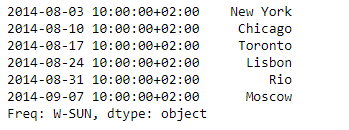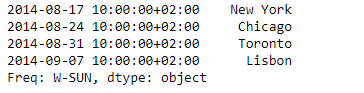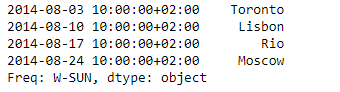Related Articles
Python | Pandas Series.slice_shift()
• Last Updated : 05 Feb, 2019

Pandas series is a One-dimensional ndarray with axis labels. The labels need not be unique but must be a hashable type. The object supports both integer- and label-based indexing and provides a host of methods for performing operations involving the index.

Pandas` Series.slice_shift()` function is equivalent to shift without copying data. The shifted data will not include the dropped periods and the shifted axis will be smaller than the original.

Syntax: Series.slice_shift(periods=1, axis=0)

Parameter :
periods : Number of periods to move, can be positive or negative

Returns : shifted : same type as caller

Example #1: Use `Series.slice_shift()` function to shift the data of the given Series object by 2 periods.

 `# importing pandas as pd ` `import` `pandas as pd ` ` `  `# Creating the Series ` `sr ``=` `pd.Series([``'New York'``, ``'Chicago'``, ``'Toronto'``, ``'Lisbon'``, ``'Rio'``, ``'Moscow'``]) ` ` `  `# Create the Datetime Index ` `didx ``=` `pd.DatetimeIndex(start ``=``'2014-08-01 10:00'``, freq ``=``'W'``,  ` `                     ``periods ``=` `6``, tz ``=` `'Europe/Berlin'``)  ` ` `  `# set the index ` `sr.index ``=` `didx ` ` `  `# Print the series ` `print``(sr) `

Output :Now we will use `Series.slice_shift()` function to shift the data in the given series object by 2 periods.

 `# shift by 2 periods ` `sr.slice_shift(periods ``=` `2``) `

Output :As we can see in the output, the `Series.slice_shift()` function has successfully shifted the data over the index. Notice the first two index labels are dropped.

Example #2: Use `Series.slice_shift()` function to shift the data of the given Series object by -2 periods.

 `# importing pandas as pd ` `import` `pandas as pd ` ` `  `# Creating the Series ` `sr ``=` `pd.Series([``'New York'``, ``'Chicago'``, ``'Toronto'``, ``'Lisbon'``, ``'Rio'``, ``'Moscow'``]) ` ` `  `# Create the Datetime Index ` `didx ``=` `pd.DatetimeIndex(start ``=``'2014-08-01 10:00'``, freq ``=``'W'``,  ` `                     ``periods ``=` `6``, tz ``=` `'Europe/Berlin'``)  ` ` `  `# set the index ` `sr.index ``=` `didx ` ` `  `# Print the series ` `print``(sr) `

Output :Now we will use `Series.slice_shift()` function to shift the data in the given series object by -2 periods.

 `# shift by -2 periods ` `sr.slice_shift(periods ``=` `-``2``) `

Output :As we can see in the output, the `Series.slice_shift()` function has successfully shifted the data over the index. Notice the last two index labels are dropped and the data has been shifted upward.

Attention geek! Strengthen your foundations with the Python Programming Foundation Course and learn the basics.

To begin with, your interview preparations Enhance your Data Structures concepts with the Python DS Course.

My Personal Notes arrow_drop_up
Recommended Articles
Page :